# HSSlive: Plus One & Plus Two Notes & Solutions for Kerala State Board

## AP Board Class 7 Maths Chapter 6 Ratio Applications Ex 3 Textbook Solutions PDF: Download Andhra Pradesh Board STD 7th Maths Chapter 6 Ratio Applications Ex 3 Book AnswersAP Board Class 7 Maths Chapter 6 Ratio Applications Ex 3 Textbook Solutions PDF: Download Andhra Pradesh Board STD 7th Maths Chapter 6 Ratio Applications Ex 3 Book Answers

## Andhra Pradesh State Board Class 7th Maths Chapter 6 Ratio Applications Ex 3 Books Solutions

 Board AP Board Materials Textbook Solutions/Guide Format DOC/PDF Class 7th Subject Maths Chapters Maths Chapter 6 Ratio Applications Ex 3 Provider Hsslive

2. Click on the Andhra Pradesh Board Class 7th Maths Chapter 6 Ratio Applications Ex 3 Answers.
3. Look for your Andhra Pradesh Board STD 7th Maths Chapter 6 Ratio Applications Ex 3 Textbooks PDF.
4. Now download or read the Andhra Pradesh Board Class 7th Maths Chapter 6 Ratio Applications Ex 3 Textbook Solutions for PDF Free.

## AP Board Class 7th Maths Chapter 6 Ratio Applications Ex 3 Textbooks Solutions with Answer PDF Download

Find below the list of all AP Board Class 7th Maths Chapter 6 Ratio Applications Ex 3 Textbook Solutions for PDF’s for you to download and prepare for the upcoming exams:

Question 1.
A length of a bacteria enlarged 50,000 times attains a length of 5 cm. What is the actual length of the bacteria? If the length is enlarged 20,000 times only, what would be its enlarged length?
Solution:
After enlarging 50,000 times the length is 5 cm.
Without enlarging (1 – times) the length Is x cm say
∴ 50,000 : 5 :: 1 : x
By rule of properties
50,000 × x = 5 × 1
x = 550,000=110,000 = 0.0001 cm

Question 2.
Observe the following tables and fmd if x is directly proportional.
Solution:
(i)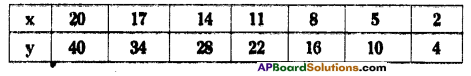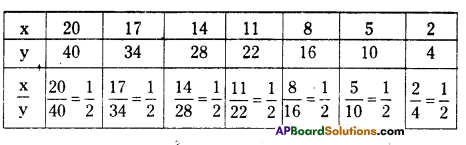In all the cases 𝑥𝑦 is constant.
∴ x ∝ y (x is directly proportional toy)

(ii)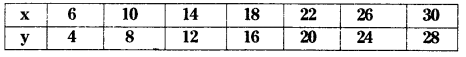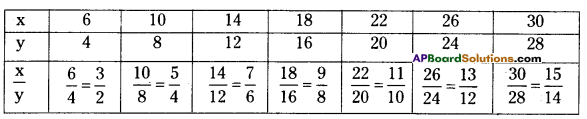Here the change/increase is not uniform.
(i.e.,) 𝑥𝑦 is not same in all cases.
∴ x is not directly proportional to y.

(iii)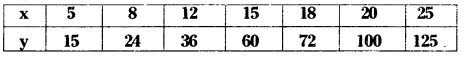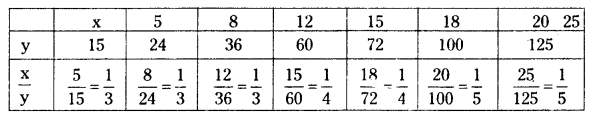Here the change/increase is not uniform.
(i.e.,) 𝑥𝑦 is not same in all cases.
∴ x is not directly proportional to y.

Question 3.
Sushma has a road map with a scale of 1 cm representing 18 km. She drives on a road for 72 km. What would be her distance covered in the map?
Solution:
Scale of the map is 1 cm = 18 km
Distance covered = 72 km
Let it be xcm on the map
then 1 : 18 :: x : 72
By rule of proportion
Product of extremes Product oÍ means
18x = 72 × 1
x = 7218 = 4cm

Question 4.
On a Grid paper, draw five squares of different sizes. Write the following information in a tabular form.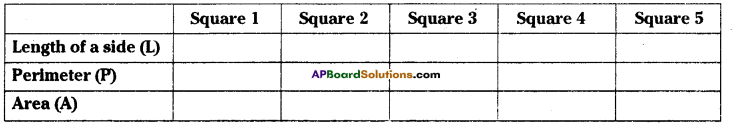Find whether the length of a side is in direct proportion to:
(i) the perimeter of the square.
(ii) the area of the square.
Solution: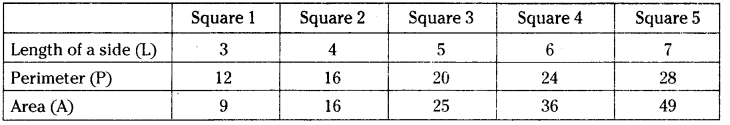## Andhra Pradesh Board Class 7th Maths Chapter 6 Ratio Applications Ex 3 Textbooks for Exam Preparations

Andhra Pradesh Board Class 7th Maths Chapter 6 Ratio Applications Ex 3 Textbook Solutions can be of great help in your Andhra Pradesh Board Class 7th Maths Chapter 6 Ratio Applications Ex 3 exam preparation. The AP Board STD 7th Maths Chapter 6 Ratio Applications Ex 3 Textbooks study material, used with the English medium textbooks, can help you complete the entire Class 7th Maths Chapter 6 Ratio Applications Ex 3 Books State Board syllabus with maximum efficiency.

## FAQs Regarding Andhra Pradesh Board Class 7th Maths Chapter 6 Ratio Applications Ex 3 Textbook Solutions

#### Can we get a Andhra Pradesh State Board Book PDF for all Classes?

Yes you can get Andhra Pradesh Board Text Book PDF for all classes using the links provided in the above article.

## Important Terms

Andhra Pradesh Board Class 7th Maths Chapter 6 Ratio Applications Ex 3, AP Board Class 7th Maths Chapter 6 Ratio Applications Ex 3 Textbooks, Andhra Pradesh State Board Class 7th Maths Chapter 6 Ratio Applications Ex 3, Andhra Pradesh State Board Class 7th Maths Chapter 6 Ratio Applications Ex 3 Textbook solutions, AP Board Class 7th Maths Chapter 6 Ratio Applications Ex 3 Textbooks Solutions, Andhra Pradesh Board STD 7th Maths Chapter 6 Ratio Applications Ex 3, AP Board STD 7th Maths Chapter 6 Ratio Applications Ex 3 Textbooks, Andhra Pradesh State Board STD 7th Maths Chapter 6 Ratio Applications Ex 3, Andhra Pradesh State Board STD 7th Maths Chapter 6 Ratio Applications Ex 3 Textbook solutions, AP Board STD 7th Maths Chapter 6 Ratio Applications Ex 3 Textbooks Solutions,
Share: